# A generalized groundwater flow equation using the concept of variable-order derivative

## Abstract

In this paper, the groundwater flow equation is generalized using the concept of the variational order derivative. We present a numerical solution of the modified groundwater flow equation with the variational order derivative. We solve the generalized equation with the Crank-Nicholson technique. Numerical methods typically yield approximate solutions to the governing equation through the discretization of space and time and can relax the rigid idealized conditions of analytical models or lumped-parameter models. They can therefore be more realistic and flexible for simulating field conditions. Within the discredited problem domain, the variable internal properties, boundaries, and stresses of the system are approximated. We perform the stability and convergence analysis of the Crank-Nicholson method and complete the paper with some illustrative computational examples and their simulations.

## 1 Introduction

A problem that arises naturally in groundwater investigations is to choose an appropriate geometry for the geological system in which the flow occurs. This geological formation, through which the groundwater flows, changes in time and space. Figure 1 shows the groundwater flow direction toward the pumping well during the extraction of water from the aquifer. The main effort is to establish a suitable mathematical relation between different observables, or what  calls a mathematical model for the observables. An ideal mathematical model should not merely provide links between different observables, but also lead to a better understanding of the phenomenon. The attractiveness of this approach is that the mathematical model could, in principle, be used to investigate the future behavior of a given phenomenon under various conditions. This leads us to the groundwater flow equation. This model is based on the conventional, saturated groundwater flow equation for density-independent flow:

${S}_{0}\left(\underline{x},t\right){\partial }_{t}\mathrm{\Phi }\left(\underline{x},t\right)=\mathrm{\nabla }\cdot \left[\underline{\underline{K}}\mathrm{\nabla }\mathrm{\Phi }\right]+f\left(x,t\right).$
(1.1)

Here ${S}_{0}$ is the specific storativity, $\underline{\underline{K}}$ is the hydraulic conductivity tensor of the aquifer, Φ is the piezometric head, $f\left(x,t\right)$ is the strength of any sources or sinks. As a review of the derivation of Eq. (1.1), we will show the Darcy law

$q\left(x,t\right)=\underline{\underline{K}}\left[\mathrm{\Phi }\left(x,t\right)\right]$
(1.2)

is used as a keystone in the derivation of Eq. (1.1). This law, proposed by Darcy early in the nineteenth century, relies on experimental results obtained from the flow of water through a one-dimensional sand column. Alternatively, Darcy’s law states that the rate of flow through a porous medium is proportional to the loss of head and inversely proportional to the length of the flow path. Note that the specific discharge $q\left(x,t\right)$ has the dimensions of velocity. Recent investigations  suggest that the flow is also influenced by the geometry of the bedding parallel fractures, the feature that equation (1.1) cannot account for. It is therefore possible that equation (1.1) may not be applicable to the flow in these fractured aquifers. In an attempt to circumvent this problem, Barker  introduced the model in which the geometry of the aquifer is regarded as a fractal. Although this model has been applied with reasonable success in the analysis of hydraulic tests from boreholes in Karoo aquifers , it introduces parameters for which no sound definition exists in the case of non-integer flow dimensions. Recently [5, 6], the concept of a fractional-order derivative was used to generalize the groundwater flow equation. However, it has been found that the constant-order fractional diffusion equations are not capable of characterizing some complex diffusion processes, for instance, diffusion process in aninhomogeneous or heterogeneous medium .

In addition, when we consider the diffusion process in a porous medium, if the medium structure or external field changes with time, in this situation, the constant-order fractional diffusion equation model cannot be used to well characterize such a phenomenon . This is the case of the groundwater flow problem, the medium through which the flow occurs is heterogeneous and changes with time. Still in some biology diffusion processes, the concentration of particles will determine the diffusion pattern [10, 11]. To solve the above problems, the variable-order (VO) fractional diffusion equation models have been suggested for use . This present work is therefore devoted to the discussion underpinning the description of the groundwater flow equation with the variable-order derivative.

## 2 Modified groundwater flow equation

For the readers that are not acquainted with the concept of the variational order derivative, we start this section by presenting the basic definition of this derivative.

### 2.1 Variational order differential operator

Let $f:R\to R$, $x\to f\left(x\right)$ denote a continuous and necessary differentiable, let $\alpha \left(x\right)$ be a continuous function in $\left(0,1\right]$. Then its variational order differential in $\left[a,\mathrm{\infty }\right)$ is defined as

${D}_{0}^{\alpha \left(x\right)}\left(f\left(x\right)\right)=\frac{1}{\mathrm{\Gamma }\left(1-\alpha \left(x\right)\right)}{\int }_{0}^{x}{\left(x-t\right)}^{-\alpha \left(t\right)}\frac{df\left(t\right)}{dt}\phantom{\rule{0.2em}{0ex}}dt.$
(2.1)

The above derivative is called the Caputo variational order differential operator; in addition, the derivative of the constant is zero.

### 2.2 Problem formulation

Groundwater models describe the groundwater flow and transport processes using mathematical equations based on certain simplifying assumptions. These assumptions typically involve the direction of flow, geometry of the aquifer, the heterogeneity or anisotropy of sediments or bedrock within the aquifer. This geological formation, through which the groundwater flows, changes in time and space.

The simplest generalization of the groundwater flow equation, which incidentally is also in accord with true physics of the phenomenon, is to assume that water level is not in a steady but transient state. Theis (1935)  was the first to develop a formula for unsteady-state flow that introduces the time factor and the storativity. He noted that when a well penetrating an extensive confined aquifer is pumped at a constant rate, the influence of the discharge extends outward with time. The rate of decline of head multiplied by the storativity and summed over the area of influence equals the discharge. The unsteady-state (or Theis) equation, which was derived from the analogy between the flow of groundwater and the conduction of heat, is perhaps the most widely used partial differential equation in groundwater investigations

$S{D}_{t}\mathrm{\Phi }\left(r,t\right)=T{D}_{rr}\mathrm{\Phi }\left(r,t\right)+\frac{1}{r}{D}_{r}\mathrm{\Phi }\left(r,t\right).$
(2.2)

The above equation is classified under a parabolic equation. To include explicitly the variability of the medium through which the flow takes place, the standard version of the partial derivative with respect to time is replaced here with variable-order (VO) fractional to obtain

$\left\{\begin{array}{c}S{D}_{t}^{\alpha \left(x,t\right)}\mathrm{\Phi }\left(r,t\right)=T{D}_{rr}\mathrm{\Phi }\left(r,t\right)+\frac{1}{r}{D}_{r}\mathrm{\Phi }\left(r,t\right),\hfill \\ 0<\alpha \left(x,t\right)\le 1.\hfill \end{array}$
(2.3)

## 3 Numerical solution

Environmental phenomena such as groundwater flow described by variational order derivative are highly complex phenomena, which do not lend themselves readily to the analysis of analytical models. The discussion presented in this section will therefore be devoted to the derivation of a numerical solution to groundwater flow equation (2.3).

Numerical methods yield approximate solutions to the governing equation through the discretization of space and time. Within the discredited problem domain, the variable internal properties, boundaries, and stresses of the system are approximated. Deterministic, distributed-parameter, numerical models can relax the rigid idealized conditions of analytical models or lumped-parameter models, and they can therefore be more realistic and flexible for simulating field conditions. The finite difference schemes for constant-order time or space fractional diffusion equations have been widely studied . For constant-order time fractional diffusion equations, Chen et al. proposed an implicit difference approximation scheme . Yuste et al. introduced weighted average finite difference methods . Podlubny et al. proposed the matrix approach for fractional diffusion equations , and Hanert proposed a flexible numerical scheme for the discretization of the space-time fractional diffusion equation . Recently, Zhuang et al. considered the numerical schemes for VO space fractional advection-dispersion equation , Lin et al. investigated the explicit scheme for VO nonlinear space fractional diffusion equation . Before applying the numerical methods, we assume Eq. (2.3) has a unique and sufficiently smooth solution. To establish the numerical schemes for the above equation, we let ${x}_{l}=lh$, $0\le l\le M$, $Mh=L$, ${t}_{k}=k\tau$, $0\le k\le N$, $N\tau =T$, h is the step and τ is the time size, M and N are grid points.

### 3.1 Crank-Nicholson scheme 

We introduce the Crank-Nicholson scheme as follows. Firstly, the discretization of first- and second-order space derivative is stated as follows: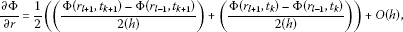(3.1)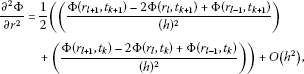(3.2)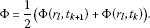(3.3)

The Crank-Nicholson scheme for the VO time fractional groundwater flow model can be stated as follows:

$\begin{array}{rcl}\frac{{\partial }^{{\alpha }_{l}^{k+1}}\mathrm{\Phi }\left({r}_{l},{t}_{k+1}\right)}{\partial {t}^{{\alpha }_{l}^{k+1}}}& =& \frac{{\tau }^{-{\alpha }_{l}^{k+1}}}{\mathrm{\Gamma }\left(2-{\alpha }_{l}^{k+1}\right)}\left(\mathrm{\Phi }\left({r}_{l},{t}_{k+1}\right)-\mathrm{\Phi }\left({r}_{l},{t}_{k}\right)\\ +\sum _{j=1}^{k}\left[\mathrm{\Phi }\left({r}_{l},{t}_{k+1-j}\right)-\mathrm{\Phi }\left({r}_{l},{t}_{k-j}\right)\right]\left[{\left(j+1\right)}^{1-{\alpha }_{l}^{k+1}}-{\left(j\right)}^{1-{\alpha }_{l}^{k+1}}\right]\right).\end{array}$
(3.4)

Now, replacing equations (3.1), (3.2), (3.3) and (3.4) in (2.3), we obtain the following:

$\begin{array}{c}\left[\frac{S{\tau }^{-{\alpha }_{l}^{k+1}}}{\mathrm{\Gamma }\left(2-{\alpha }_{l}^{k+1}\right)}\left(\mathrm{\Phi }\left({r}_{l},{t}_{k+1}\right)-\mathrm{\Phi }\left({r}_{l},{t}_{k}\right)\hfill \\ \phantom{\rule{2em}{0ex}}+\sum _{j=1}^{k}\left[\mathrm{\Phi }\left({r}_{l},{t}_{k+1-j}\right)-\mathrm{\Phi }\left({r}_{l},{t}_{k-j}\right)\right]\left[{\left(j+1\right)}^{1-{\alpha }_{l}^{k+1}}-{\left(j\right)}^{1-{\alpha }_{l}^{k+1}}\right]\right)\right]\hfill \\ \phantom{\rule{1em}{0ex}}=T\left[\frac{1}{2}\left(\left(\frac{\mathrm{\Phi }\left({r}_{l+1},{t}_{k+1}\right)-2\mathrm{\Phi }\left({r}_{l},{t}_{k+1}\right)+\mathrm{\Phi }\left({r}_{l-1},{t}_{k+1}\right)}{{\left(h\right)}^{2}}\right)\hfill \\ \phantom{\rule{2em}{0ex}}+\left(\frac{\mathrm{\Phi }\left({r}_{l+1},{t}_{k}\right)-2\mathrm{\Phi }\left({r}_{l},{t}_{k}\right)+\mathrm{\Phi }\left({r}_{l-1},{t}_{k}\right)}{{\left(h\right)}^{2}}\right)\right)\right]\hfill \\ \phantom{\rule{2em}{0ex}}+\frac{1}{{r}_{l}}\left[\frac{1}{2}\left(\left(\frac{\mathrm{\Phi }\left({r}_{l+1},{t}_{k+1}\right)-\mathrm{\Phi }\left({r}_{l-1},{t}_{k+1}\right)}{2\left(h\right)}\right)+\left(\frac{\mathrm{\Phi }\left({r}_{l+1},{t}_{k}\right)-\mathrm{\Phi }\left({r}_{l-1},{t}_{k}\right)}{2\left(h\right)}\right)\right)\right].\hfill \end{array}$

For simplicity, let us put:

$\begin{array}{r}{b}_{j}^{l,k+1}={\left(j+1\right)}^{1-{\alpha }_{l}^{k+1}}-{\left(j\right)}^{1-{\alpha }_{l}^{k+1}};\phantom{\rule{2em}{0ex}}{T}_{l}^{k+1}=\frac{\mathrm{\Gamma }\left(2-{\alpha }_{l}^{k+1}\right){\tau }^{{\alpha }_{l}^{k+1}}}{S{h}^{2}}T;\\ {G}_{l}^{k+1}=\frac{\mathrm{\Gamma }\left(2-{\alpha }_{l}^{k+1}\right){\tau }^{{\alpha }_{l}^{k+1}}}{Sh}\phantom{\rule{1em}{0ex}}\text{and}\phantom{\rule{1em}{0ex}}{\lambda }_{j}^{l,k+1}={b}_{j-1}^{l,k+1}-{b}_{j}^{l,k+1}.\end{array}$
(3.5)

Equation (3.5) becomes

$\begin{array}{rcl}{\mathrm{\Phi }}_{l}^{k+1}\left(1+2{T}_{l}^{k+1}\right)& =& {\mathrm{\Phi }}_{l+1}^{k+1}\left({T}_{l}^{k+1}+\frac{{G}_{l}^{k+1}}{{r}_{l}}\right)+{\mathrm{\Phi }}_{l-1}^{k+1}\left({T}_{l}^{k+1}-\frac{{G}_{l}^{k+1}}{{r}_{l}}\right)\\ +{\mathrm{\Phi }}_{l+1}^{k}\left({T}_{l}^{k+1}-\frac{{G}_{l}^{k+1}}{{r}_{l}}\right)+{\mathrm{\Phi }}_{l}^{k+1}\left(1+2{T}_{l}^{k+1}\right)\\ +\sum _{j=1}^{k}\left({\mathrm{\Phi }}_{l}^{k+1-j}-{\mathrm{\Phi }}_{l}^{k-j}\right){\lambda }_{j}^{l,k+1}{G}_{l}^{k+1}.\end{array}$
(3.6)

## 4 Stability analysis of the Crank-Nicholson scheme

In this section, we will analyze the stability conditions of the Crank-Nicholson scheme for the generalized groundwater flow equation.

Let ${\zeta }_{l}^{k}={\mathrm{\Phi }}_{l}^{k}-{\mathrm{\Theta }}_{l}^{k}$. Here ${\mathrm{\Theta }}_{l}^{k}$ is the approximate solution at the point $\left({x}_{l},{t}_{k}\right)$ ($k=1,2,\dots ,N$, $l=1,2,\dots ,M-1$) and, in addition, ${\zeta }^{k}={\left[{\zeta }_{1}^{k},{\zeta }_{2}^{k},\dots ,{\zeta }_{M-1}^{k}\right]}^{T}$ and the function ${\zeta }^{k}\left(x\right)$ is chosen to be

(4.1)

Then the function ${\zeta }^{k}\left(x\right)$ can be expressed in Fourier series as follows:

$\begin{array}{r}{\zeta }^{k}\left(x\right)=\sum _{m=-\mathrm{\infty }}^{m=\mathrm{\infty }}{\delta }_{m}\left(m\right)exp\left[2i\pi mk/L\right],\\ {\delta }_{k}\left(x\right)=\frac{1}{L}{\int }_{0}^{L}{\rho }^{k}\left(x\right)exp\left[\frac{2i\pi mx}{L}\right]\phantom{\rule{0.2em}{0ex}}dx.\end{array}$
(4.2)

It was established by Chen et al.  that

${\parallel {\rho }^{2}\parallel }_{2}^{2}=\sum _{m=-\mathrm{\infty }}^{m=\mathrm{\infty }}{\parallel {\delta }_{k}\left(m\right)\parallel }^{2}.$
(4.3)

Observe that for all $k,l\ge 1$, $0\le 1-{\alpha }_{l}^{k+1}<1$, in addition, according to the problem in point, the storativity S, and transmissivity T are positive constants. Then the following properties of the coefficients ${T}_{l}^{k+1}$, ${G}_{l}^{k+1}$, ${\lambda }_{j}^{l,k+1}$, and ${b}_{l}^{k+1}$ can be established:

(4.4)

It is customary in groundwater investigations to choose a point on the centerline of the pumped borehole as a reference for the observations, and therefore neither the drawdown nor its derivatives will vanish at the origin, as required. In such situations where the distribution of the piezometric head in the aquifer is a decreasing function of the distance from the borehole, the expression $\frac{1}{{r}_{l}}\to 0$. Under this situation, the error committed while approximating the solution of the generalized groundwater flow equation with the Crank-Nicholson scheme can be presented as follows:

$\begin{array}{rcl}{\zeta }_{l}^{k+1}\left(1+2{T}_{l}^{k+1}\right)& =& {\zeta }_{l+1}^{k+1}\left({T}_{l}^{k+1}\right)+{\zeta }_{l-1}^{k+1}\left({T}_{l}^{k+1}\right)+{\zeta }_{l+1}^{k}\left({T}_{l}^{k+1}\right)+{\zeta }_{l}^{k}\left(1+2{T}_{l}^{k+1}\right)\\ +\sum _{j=1}^{k}\left({\zeta }_{l}^{k+1-j}-{\zeta }_{l}^{k-j}\right){\lambda }_{j}^{l,k+1}{G}_{l}^{k+1}.\end{array}$
(4.5a)

If we assume that ${\zeta }_{l}^{k}$ in equation (4.1) can be put in the delta-exponential form as follows:

${\zeta }_{l}^{k}={\delta }_{k}exp\left[i\varphi lk\right],$
(4.5b)

where ϕ is a real spatial wave number, new replacing the above equation (4.5b) into (4.5a), we obtain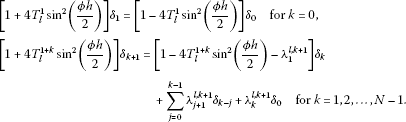(4.6)

Equation (4.6) can be written in the following form:

$\begin{array}{r}{\delta }_{1}=\frac{\left[1-4{T}_{l}^{1}{sin}^{2}\left(\frac{\varphi h}{2}\right)\right]{\delta }_{0}}{\left[1+4{T}_{l}^{1}{sin}^{2}\left(\frac{\varphi h}{2}\right)\right]},\\ {\delta }_{k+1}=\frac{\left[1-4{T}_{l}^{1+k}{sin}^{2}\left(\frac{\varphi h}{2}\right)-{e}_{1}^{l,k+1}\right]{\delta }_{k}+{\sum }_{j=0}^{k-1}{\lambda }_{j+1}^{l,k+1}{\delta }_{k-j}+{\lambda }_{k}^{l,k+1}{\delta }_{0}}{\left[1+4{T}_{l}^{1+k}{sin}^{2}\left(\frac{\varphi h}{2}\right)\right]}.\end{array}$
(4.7)

Our next concern here is to show that for all $k=1,2,\dots ,N-1$, the solution of equation (4.7) satisfies the following condition:

$|{\delta }_{k}|<|{\delta }_{0}|.$

To achieve this, we make use of the recurrence technique on the natural number k.

For $k=1$ and remembering that ${d}_{l}^{k+1}$, ${b}_{l}^{k+1}$ are positive for all $l=1,2,\dots ,M-1$, we obtain

$\frac{|{\delta }_{1}|}{|{\delta }_{0}|}=|\frac{\left[1-4{T}_{l}^{1}{sin}^{2}\left(\frac{\varphi h}{2}\right)\right]}{\left[1+4{T}_{l}^{1}{sin}^{2}\left(\frac{\varphi h}{2}\right)\right]}|<1.$
(4.8)

Assuming that for $m=2,3,\dots ,k$ the property is verified, we get

$|{\delta }_{k+1}|=|\frac{\left[1-4{T}_{l}^{1+k}{sin}^{2}\left(\frac{\varphi h}{2}\right)-{e}_{1}^{l,k+1}\right]{\delta }_{k}+{\sum }_{j=0}^{k-1}{\lambda }_{j+1}^{l,k+1}{\delta }_{k-j}+{\lambda }_{k}^{l,k+1}{\delta }_{0}}{\left[1+4{T}_{l}^{1+k}{sin}^{2}\left(\frac{\varphi h}{2}\right)\right]}|.$
(4.9)

Making use of the triangular inequality we obtain

$|{\delta }_{k+1}|\le \frac{|1-4{T}_{l}^{1+k}{sin}^{2}\left(\frac{\varphi h}{2}\right)-{e}_{1}^{l,k+1}||{\delta }_{k}|+|{\sum }_{j=0}^{k-1}{p}_{j+1}^{l,k+1}{\delta }_{k-j}|+|{e}_{k}^{l,k+1}{\delta }_{0}|}{|1+4{T}_{l}^{1+k}{sin}^{2}\left(\frac{\varphi h}{2}\right)|}.$
(4.10)

Using the recurrence hypothesis, we have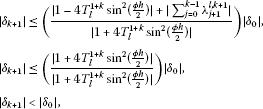(4.11)

which this completes the proof.

## 5 Convergence analysis of the Crank-Nicholson scheme

If we assume that $\mathrm{\Phi }\left({r}_{l},{t}_{k}\right)$ ($l=1,2,\dots ,M$, $k=1,2,\dots ,N-1$) is the exact solution of our problem at the point $\left({r}_{l},{t}_{k}\right)$, by letting ${\mathrm{\Omega }}_{l}^{k}=\mathrm{\Phi }\left({r}_{l},{t}_{k}\right)-{\mathrm{\Phi }}_{l}^{k}$ and ${\mathrm{\Omega }}^{k}=\left(0,{\mathrm{\Omega }}_{1}^{k},{\mathrm{\Omega }}_{2}^{k},\dots ,{\mathrm{\Omega }}_{M-1}^{k}\right)$, substituting this in equation (4.7), we obtain

(5.1)

Here,

$\begin{array}{rcl}{R}_{l}^{1+k}& =& \mathrm{\Phi }\left({r}_{l},{t}_{k+1}\right)-\sum _{j=0}^{k-1}\mathrm{\Phi }\left({r}_{l},{t}_{k-j}\right){\lambda }_{j+1}^{l,k+1}+{b}_{1}^{l,k+1}\mathrm{\Phi }\left({r}_{l},{t}_{0}\right)\\ -{T}_{l}^{1+k}\left[\mathrm{\Phi }\left({r}_{l+1},{t}_{k+1}\right)-2\mathrm{\Phi }\left({r}_{l},{t}_{k+1}\right)+\mathrm{\Phi }\left({r}_{l-1},{t}_{k+1}\right)\right].\end{array}$
(5.2)

From equations (3.1) and (3.4), we have

$\begin{array}{c}\frac{{\partial }^{2}\mathrm{\Phi }\left({r}_{l},{t}_{k+1}\right)}{\partial {r}^{2}}+{h}^{2}{V}_{1}=\frac{1}{2}\left(\frac{\left(\mathrm{\Phi }\left({r}_{l+1},{t}_{k+1}\right)-2\mathrm{\Phi }\left({r}_{l},{t}_{k+1}\right)+\mathrm{\Phi }\left({r}_{l-1},{t}_{k+1}\right)\right)}{{h}^{2}}\hfill \\ \phantom{\frac{{\partial }^{2}\mathrm{\Phi }\left({r}_{l},{t}_{k+1}\right)}{\partial {r}^{2}}+{h}^{2}{V}_{1}=}+\frac{\left(\mathrm{\Phi }\left({r}_{l+1},{t}_{k}\right)-2\mathrm{\Phi }\left({r}_{l},{t}_{k}\right)+\mathrm{\Phi }\left({r}_{l-1},{t}_{k}\right)\right)}{{h}^{2}}\right),\hfill \\ \frac{{\partial }^{{\alpha }_{l}^{k+1}}\mathrm{\Phi }\left({r}_{l},{t}_{k+1}\right)}{\partial {t}^{{\alpha }_{l}^{k+1}}}+\tau {V}_{2}\hfill \\ \phantom{\rule{1em}{0ex}}=\frac{{\tau }^{-{\alpha }_{l}^{k+1}}}{\mathrm{\Gamma }\left(2-{\alpha }_{l}^{k+1}\right)}\left(\mathrm{\Phi }\left({r}_{l},{t}_{k+1}\right)-\mathrm{\Phi }\left({r}_{l},{t}_{k}\right)+\sum _{j=1}^{k}\left[\mathrm{\Phi }\left({r}_{l},{t}_{k+1-j}\right)-\mathrm{\Phi }\left({r}_{l},{t}_{k-j}\right)\right]{\lambda }_{j}^{l,k}\right).\hfill \end{array}$

From the above, we have that

${R}_{l}^{k+1}\le K\left({\tau }^{1+{\alpha }_{l}^{k+1}}+{h}^{2}{\tau }^{{\alpha }_{l}^{k}}\right),$
(5.3)

where ${K}_{1}$, ${K}_{2}$, and K are constants. Taking into account the Caputo-type fractional derivative, the detailed error analysis on the above schemes can refer to the work by Diethelm et al.  and further work by Li and Tao .

Lemma 1 ${\parallel {\mathrm{\Omega }}^{k+1}\parallel }_{\mathrm{\infty }}\le K\left({\tau }^{1+{\alpha }_{l}^{k+1}}+{h}^{2}{\tau }^{{\alpha }_{l}^{k}}\right){\left({\mathrm{\Omega }}_{j}^{l,k+1}\right)}^{-1}$ is true for ($k=0,1,2,\dots ,N-1$), where ${\parallel {w}^{k}\parallel }_{\mathrm{\infty }}={max}_{1\le l\le M-1}\left({\mathrm{\Omega }}^{k}\right)$, K is a constant. In addition,

${\alpha }^{k+1}=\left\{\begin{array}{cc}{min}_{1\le l\le M-1}{\alpha }_{l}^{k+1},\hfill & \mathit{\text{if}}\tau <1,\hfill \\ {max}_{1\le l\le M-1}{\alpha }_{l}^{k+1},\hfill & \mathit{\text{if}}\tau >1.\hfill \end{array}$

This can be achieved via the recurrence technique on the natural number k.

When $k=0$, we have the following:

$\begin{array}{rcl}|{\mathrm{\Omega }}_{l}^{1}|& \le & \left({c}_{l}^{1}+{b}_{l}^{1}\right)|{w}_{l+1}^{1}|+\left({c}_{l}^{1}-2{b}_{l}^{1}\right)|{w}_{l-1}^{1}|\\ =& |{F}_{l}^{1}|\le V\left({\tau }^{1+{\alpha }_{l}^{k+1}}+{h}^{2}{\tau }^{{\alpha }_{l}^{k}}\right){\left({\lambda }_{j}^{l,k+1}\right)}^{-1}.\end{array}$
(5.4)

Now suppose that ${\parallel {\mathrm{\Omega }}^{i+1}\parallel }_{\mathrm{\infty }}\le K\left({\tau }^{1+{\alpha }_{l}^{i+1}}+{h}^{2}{\tau }^{{\alpha }_{l}^{i}}\right){\left({\lambda }_{j}^{l,i+1}\right)}^{-1}$, $i=1,\dots ,N-2$. Then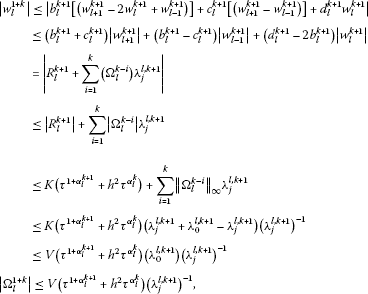(5.5)

which completes the proof.

Theorem 1 The Crank-Nicholson scheme is convergent, and there exists a positive constant V such that

$|{\mathrm{\Phi }}_{l}^{k}-\mathrm{\Phi }\left({x}_{l},{t}_{k}\right)|\le K\left(\tau +{h}^{2}\right),\phantom{\rule{1em}{0ex}}l=1,2,\dots ,M-1,k=1,2,\dots ,N.$
(5.6)

An interested reader can find the solvability of the Crank-Nicholson scheme in the work done by . Therefore, the details of the proof will not be presented in this paper.

## 6 Numerical results

An image is worth ten thousand words; therefore, we devote this section to the numerical simulations of the solution of the generalized groundwater flow equation. The parameters used in the simulation are given as $S=0.0086$, $T=400$, $r\in \left[0.5,35\right]$, and $t\in \left[0,10\right]$.

Example

$\left\{\begin{array}{c}S{D}_{t}^{\alpha \left(x,t\right)}\mathrm{\Phi }\left(r,t\right)=T{D}_{rr}\mathrm{\Phi }\left(r,t\right)+\frac{1}{r}{D}_{r}\mathrm{\Phi }\left(r,t\right),\hfill \\ \alpha \left(x,t\right)=1-sin\left(rt\right),\hfill \\ \mathrm{\Phi }\left(r,0\right)=2.\hfill \end{array}$
(6.1)

The numerical simulations of the approximate solution are presented in figures. First, Figure 2 shows the solution as a function of time and space. Figure 3 shows the solution as a function of time for a fixed distance. Figure 4 shows the solution as a function of space for fixed time.

## 7 Conclusion

In this paper, the groundwater flow equation was generalized using the concept of a variational order derivative. The new equation was solved numerically via the Crank-Nicholson technique. We presented in detail the stability and the convergence of this problem. We presented numerical simulations.

## References

1. Botha, JF, Buys, J, Verwey, JP, Tredoux, G, Moodie, JW, Hodgkiss, M: Modelling groundwater contamination in the Atlantis aquifer. WRC Report No 175/1/90. Water Research Commission, P.O. Box 824, Pretoria 0001 (2004)

2. Van Tonder GJ, Botha JF, Chiang WH, Kunstmann H, Xu Y: Estimation of sustainable yields of boreholes in fractured rock formations. J. Hydrol. 2001, 241: 70-90. 10.1016/S0022-1694(00)00369-3

3. Barker JA: A generalised radial flow model for hydraulic tests in fractured rock. Water Resour. Res. 1988, 24(10):1796-1804. 10.1029/WR024i010p01796

4. Van Der Voort, I: Risk-based decision tools for managing and protecting groundwater resources. PhD dissertation, University of the Free State, P.O. Box 339, Bloemfontein (2001)

5. Atangana A: Numerical solution of space-time fractional order derivative of groundwater flow equation. International Conference of Algebra and Applied Analysis 2012, 20.

6. Botha JF, Cloot AH: A generalized groundwater flow equation using the concept of non-integer order. Water SA 2006, 32(1):1-7.

7. Solomon TH, Weeks ER, Swinney HL: Observation of anomalous diffusion and Lévy flights in a two-dimensional rotating flow. Phys. Rev. Lett. 1993, 71: 3975-3978. 10.1103/PhysRevLett.71.3975

8. Magin RL, Abdullah O, Baleanu D, Zhou XJ: Anomalous diffusion expressed through fractional order differential operators in the Bloch-Torrey equation. J. Magn. Reson. 2008, 190: 255-270. 10.1016/j.jmr.2007.11.007

9. Sun HG, Chen W, Chen YQ: Variable order fractional differential operators in anomalous diffusion modeling. Phys. A 2009, 388: 4586-4592. 10.1016/j.physa.2009.07.024

10. Umarov S, Steinberg S: Variable order differential equations and diffusion with changing modes. Z. Anal. Anwend. 2009, 28: 431-450.

11. Ross B, Samko SG:Fractional integration operator of variable order in the Hölder space $H\lambda \left(x\right)$. Int. J. Math. Math. Sci. 1995, 18: 777-788. 10.1155/S0161171295001001

12. Chen YQ, Moore KL: Discretization schemes for fractional-order differentiators and integrators. IEEE Trans. Circ. Syst. I: Fund. Theoret. Appl. 2002, 49: 363-367. 10.1109/81.989172

13. Theis CV: The relation between the lowering of the piezometric surface and the rate and duration of discharge of a well using groundwater storage. Trans. Am. Geophys. Union 1935, 16: 519-524. 10.1029/TR016i002p00519

14. Zhang Y: A finite difference method for fractional partial differential equation. Appl. Math. Comput. 2009, 215: 524-529. 10.1016/j.amc.2009.05.018

15. Tadjeran C, Meerschaert MM, Scheffler HP: A second order accurate numerical approximation for the fractional diffusion equation. J. Comput. Phys. 2006, 213: 205-213. 10.1016/j.jcp.2005.08.008

16. Meerschaert MM, Tadjeran C: Finite difference approximations for fractional advection dispersion equations. J. Comput. Appl. Math. 2004, 172: 65-77. 10.1016/j.cam.2004.01.033

17. Deng WH: Numerical algorithm for the time fractional Fokker-Planck equation. J. Comput. Phys. 2007, 227: 1510-1522. 10.1016/j.jcp.2007.09.015

18. Li CP, Chen A, Ye JJ: Numerical approaches to fractional calculus and fractional ordinary differential equation. J. Comput. Phys. 2011, 230: 3352-3368. 10.1016/j.jcp.2011.01.030

19. Chen CM, Liu F, Turner I, Anh V: A Fourier method for the fractional diffusion equation describing sub-diffusion. J. Comput. Phys. 2007, 227: 886-897. 10.1016/j.jcp.2007.05.012

20. Yuste SB, Acedo L: An explicit finite difference method and a new Von Neumann-type stability analysis for fractional diffusion equations. SIAM J. Numer. Anal. 2005, 42: 1862-1874. 10.1137/030602666

21. Podlubny I, Chechkin A, Skovranek T, Chen YQ, Vinagre Jara BM: Matrix approach to discrete fractional calculus II: partial fractional differential equations. J. Comput. Phys. 2009, 228: 3137-3153. 10.1016/j.jcp.2009.01.014

22. Hanert E: On the numerical solution of space time fractional diffusion models. Comput. Fluids 2011, 46: 33-39. 10.1016/j.compfluid.2010.08.010

23. Lin R, Liu F, Anh V, Turner I: Stability and convergence of a new explicit finite-difference approximation for the variable-order nonlinear fractional diffusion equation. Appl. Math. Comput. 2009, 212: 435-445. 10.1016/j.amc.2009.02.047

24. Crank J, Nicolson P: A practical method for numerical evaluation of solutions of partial differential equations of the heat conduction type. Proc. Camb. Philol. Soc. 1947, 43(1):50-67. 10.1017/S0305004100023197

25. Diethelm K, Ford NJ, Freed AD: Detailed error analysis for a fractional Adams method. Numer. Algorithms 2004, 36: 31-52.

26. Li CP, Tao CX: On the fractional Adams method. Comput. Math. Appl. 2009, 58: 1573-1588. 10.1016/j.camwa.2009.07.050

## Acknowledgements

Dedicated to Professor Hari M Srivastava.

The authors would like to thank the referee for some valuable comments and helpful suggestions. This study was supported by the National Research Fund of South Africa.

## Author information

Authors

### Corresponding author

Correspondence to Abdon Atangana.

### Competing interests

The authors declare that they have no competing interests.

### Authors’ contributions

AA wrote the first draft and FJB corrected the final version. Both authors read and approved the final draft.

## Authors’ original submitted files for images

Below are the links to the authors’ original submitted files for images.

## Rights and permissions

Reprints and Permissions

Atangana, A., Botha, J.F. A generalized groundwater flow equation using the concept of variable-order derivative. Bound Value Probl 2013, 53 (2013). https://doi.org/10.1186/1687-2770-2013-53

• Accepted:

• Published:

• DOI: https://doi.org/10.1186/1687-2770-2013-53

### Keywords

• groundwater flow equation
• variable order derivative
• Crank-Nicholson scheme
• stability
• convergence